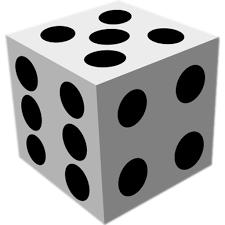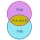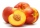# Sum or product

What is the probability that two dice fall will have the sum 7 or product 12?

p =  0.2222

### Step-by-step explanation:Did you find an error or inaccuracy? Feel free to write us. Thank you!Tips to related online calculators
Would you like to compute count of combinations?

## Related math problems and questions:

• Fall sum or sameFind the probability that if you roll two dice, it will fall the sum of 10, or the same number will fall on both dice.
• Sum on diceWe have two dice. What is the greater likelihood of fall a total sum 7 or 8 ? (write 7, 8 or 0 if the probabilities are the same)?
• Six on diceWhat is the probability that when throwing two dice will fall at least one six?
• Dices throwsWhat is the probability that the two throws of the dice: a) Six falls even once b) Six will fall at least once
• DiceWe throw five times the dice. What is the probability that six fits exactly twice?
• DiceWe throw 10 times a playing dice. What is the probability that the six will fall exactly 4 times?
• One threeWe throw two dice. What is the probability that max one three falls?
• ThrowWe throw 2 times with 2 dices. What is the probability that the first roll will fall more than sum of 9 and the second throw have sum 3 or does not have the sum 4?
• Two diceWe roll two dice. What is the probability that the sum of the falling numbers is greater than 3?
• Pairs of socksFerdinand has twelve pairs of socks, and one sock is leaky. What is the probability of putting on a leaky sock?
• ClassroomOf the 26 pupils in the classroom, 12 boys and 14 girls, four representatives are picked to the odds of being: a) all the girls b) three girls and one boy c) there will be at least two boys
• PeachesThere are 20 peaches in the pocket. 3 peaches are rotten. What is the probability that one of the randomly picked two peaches will be just one rotten?
• We rollWe roll two dice A. - what is the probability that the sum of the falling numbers is at most 4 B. - is at least 10 C. - is divisible by 5?There are 15 boys and 12 girls at the graduation party. Determine how many four couples can be selected.I have 4 sweaters two are white, 1 red and 1 green. How many ways can this done?The player throwing the three dice asked G. Galilei the question: "Should I bet on the sum of 11 or the sum of 12?" What did Galilei answer him? Hint: write down all three triples of numbers that can be thrown and: have a total of 11 have a total of 12 anWhat is the probability of events that if we throw a dice is rolled less than 6?